# CBSE Class 10 Science Magnetic Effects of Electric Current Assignment Set A

Read and download free pdf of CBSE Class 10 Science Magnetic Effects of Electric Current Assignment Set A. Get printable school Assignments for Class 10 Science. Class 10 students should practise questions and answers given here for Chapter 13 Magnetic Effect Of Electric Current Science in Class 10 which will help them to strengthen their understanding of all important topics. Students should also download free pdf of Printable Worksheets for Class 10 Science prepared as per the latest books and syllabus issued by NCERT, CBSE, KVS and do problems daily to score better marks in tests and examinations

## Assignment for Class 10 Science Chapter 13 Magnetic Effect Of Electric Current

Class 10 Science students should refer to the following printable assignment in Pdf for Chapter 13 Magnetic Effect Of Electric Current in Class 10. This test paper with questions and answers for Class 10 Science will be very useful for exams and help you to score good marks

### Chapter 13 Magnetic Effect Of Electric Current Class 10 Science Assignment

Question. When a coil carries current in an anti clockwise direction what pole does it create?
a. South pole
b. Zero pole
c. North pole
d. both South pole and North pole
Explanation: According to clock rule if we look at the face of the coil which carries current in the clock wise direction then the face is called as south pole looking from the other side of coil we find that anti clock wise current flows that is called as north pole.

Question. Which of the following involves electro magnetic induction?
a. A magnetic field exerts a force on a current- carrying wire
b. An electric current produces a magnetic field.
c. A rod is charged with electricity
d. The relative motion between a magnet and a coil produces an electric current.
Explanation: When a straight coil and a magnet are moved relative to each other, a current is induced in the coil. This phenomenon is known as electromagnetic induction.

True/False

Question. An electron and a proton move in a uniform magnetic field with same speed perpendicular to the magnetic field. They experience forces in opposite directions differing by a factor of 1840.

Question. The direction of force on a current carrying conductor placed in a magnetic field can be reversed by reversing the direction of current flowing in the conductor.

Question. The field lines inside the infinite solenoid are in the form of parallel straight lines.

Question. The pattern of the magnetic field around a conductor due to an electric current flowing through it depends on the shape of the conductor.

Question. The magnitude of induced current can be increased by decreasing the speed of rotation of coil.

Question. The electrician must always follow the correct colour code while wiring the household circuits.

Question. The field at the centre of a long circular coil carrying current will be parallel straight lines.

Question. It is standard practice to connect fuse wire in the neutral wire of the household wiring.

Question. We use the ‘right hand thumb rule’ for finding the direction of the magnetic field due to both a (current carrying) straight wire as well as a circular coil.

Question. Every household circuit must have proper earth wire installed in it.

Assertion and Reason

DIRECTION : In the following questions, a statement of assertion (A) is followed by a statement of reason (R). Mark the correct choice as:
(a) Both assertion (A) and reason (R) are true and reason (R) is the correct explanation of assertion (A).
(b) Both assertion (A) and reason (R) are true but reason (R) is not the correct explanation of assertion (A).
(c) Assertion (A) is true but reason (R) is false.
(d) Assertion (A) is false but reason (R) is true.
(e) Both Assertion and Reason are false.

Question. Assertion : A neutral body may experience a net nonzero magnetic force.
Reason : The net charge on a current carrying wire is zero, but it can experience a force in a magnetic field.

Question. Assertion : When number of turns in a coil is doubled coefficient of self-inductance of the coil becomes 4 times.
Reason : This is because L ? N2 .

Question. Assertion : A compass needle is placed near a current carrying wire. The deflection of the compass needle decreases when the compass needle is displaced away from the wire.
Reason : Strength of a magnetic field decreases as one moves away from a current carrying conductor.

Question. Assertion : In Fleming’s Left Hand Rule, the direction of magnetic field, force and current are mutually perpendicular.
Reason : Fleming’s Left hand Rule is applied to measure the induced current.

Question. Assertion : A small coil carrying current, in equilibrium, is perpendicular to the direction of the uniform magnetic field.
Reason : Torque is maximum when plane of coil and direction of the magnetic field are parallel to each other.

Question. Assertion : On changing the direction of flow of current through a straight conductor, the direction of a magnetic field around the conductor is reversed.
Reason : The direction of magnetic field around a conductor can be given in accordance with left hand thumb rule.

Question. Assertion : an induced current has a direction such that the magnetic flux that induces the current.
Reason : Above statement is in accordance with conservation of energy.

Question. Assertion : A proton moves horizontally towards a vertical long conductor having an upward electric current. It will deflect vertically downward.
Reason : Seeing the proton and the conductor from the side of the proton, the magnetic field at the site of the proton will be towards right. Hence the force Fv = qnv #Bv will deflect the proton vertically downward.

Question. Assertion : No net force acts on a rectangular coil carrying a steady current when suspended freely in a uniform magnetic field.
Reason : Force on coil in magnetic field is always nonzero.

Question. Assertion : The strength of the magnetic field produced at the centre of a current carrying circular coil increases on increasing the radius of the circular coil.
Reason : Magnetic field strength is inversely proportional to the radius of the circular coil.

Question. Assertion : Lenz’s law violates the principle of conservation of energy.
Reason : Induced e.m.f. always opposes the change in magnetic flux responsible for its production.

Question. Assertion : Alternating Current is used in household supply.
Reason : AC electric power can be transmitted over long distance without much loss of energy.

Question. Assertion : The strength of the magnetic field produced at the centre of a current carrying circular coil increases on increasing the number of turns of the circular coil.
Reason : Magnetic field strength is directly proportional to the number of turns of the circular coil.

Matching Questions

DIRECTION : Each question contains statements given in two columns which have to be matched. Statements (A, B, C, D) in column I have to be matched with statements (p, q, r, s) in column II.

Question.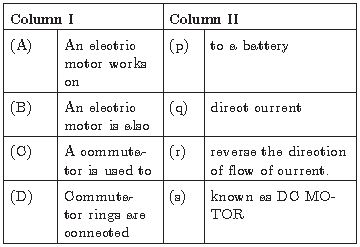Answer : A-q, B-s, C-r, D-p

Question. Column II gives approximate values of magnetic fields due to source given in column I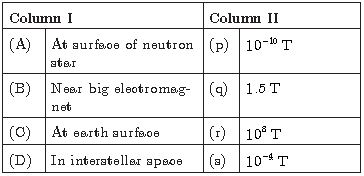Answer : A-r, B-q, C-s, D-p

Question. Equal currents i flow in two wires along x and y axis as shown. Match the following :Answer : A-r, B-q, C-r, D-p

Question. In column I, the position of small current carrying loops have been shown and in column II information related to force experienced by coil is given. Match the entries of column I with the entries of column II.(Assume solenoid radius to be small as compared to its length)Answer : (c) A-r, B-p, C-p, D-s

Question. In magnetic field, for a charged particle, match the entries of column I with the entries of column II.Answer : (a) A-p, B-r, C-s, -D-s

Question. Two wires each carrying a steady current I are shown in four configurations in Column I. Some of the resulting effects are described in Column II. Match the statements in Column I with the statements in column II.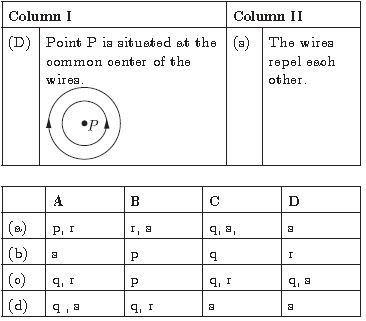Answer : (c) A-q, r B-p, C-q, r, D-q, s

Question. Column I contains some features of AC supply in India and Column II contains their relevant values/details. Match Columns I and II.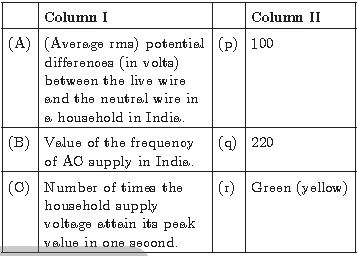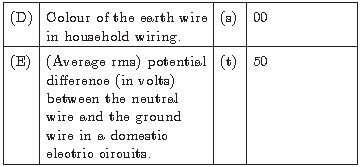Answer : A-q, B-t, C-p, D-r, E-s

Question. What does the divergence of magnetic field lines near the ends of a current carrying straight solenoid indicate?
Answer :  The divergence of magnetic field lines indicates the decrease in strength of the magnetic field near the ends of the solenoid.

Question. Why is an alternating current considered to be advantageous over direct current for long range transmission of electric energy?
Answer :  Alternating current can be transmitted without much loss of electric energy to the distant places. So AC is considered to be advantageous over DC.

Question. i. Two circular coils P and Q are kept close to each other, of which, coil P carries a current. Will some current be induced in coil Q if coil P is moved towards Q ? Give a reason for your answer and name the phenomenon involved.
ii. What happens, if coil P is moved away from Q?
iii. State few methods of inducing current in a coil.
Answer :  i. When coil P is moved towards Q, then current will be induced in coil Q because the coil P will carry a magnetic field around it, so when it is moved towards coil Q it increases the magnetic flux around it, hence, current is induced. This phenomenon is called electromagnetic induction.
ii. If P is moved away from Q, then the magnetic flux around Q will decrease and a current will be induced but in the opposite direction.
iii. Some of the methods of inducing current in the coil are as below:
a. Moving a magnet away or towards the coil.
b. Moving a coil away or towards a magnet.
c. Rotating a coil within a magnetic field.
d. By changing the magnitude of current.

Question. A current carrying conductor is placed perpendicular to the uniform magnetic field.
What happens to displacement of the conductor if
(i) Amount of current increases
(ii) If horse shoe magnet is replaced by a weak horse shoe magnet.
Answer :  The displacement of the conductor
(i) will increase on increasing the current
(ii) Will decrease on using a weak horse shoe magnet.

Question. What is the pattern of magnetic field due to a circular coil carrying current?
Answer :  Magnetic field due to circular conductor: When a current is passed through a circular coil in clockwise direction, the magnetic field will be as shown in figure.Here the current is passing clockwise when looked from front side. At the points where the conductor passes through horizontal plane, the lines of force are almost circular, their direction being given by right-hand grip rule. Near the central region, the lines are quite straight and at right angles to the plane of the coil.
Further, it is clear from the figure that all the lines enter at the nearer face and leave at the farther face. Evidently, the lines of force due to the current flowing in circular loop closely resemble those produced by a magnetized circular disc of steel of same boundary as that of the coil, so that one face of it is north and other a south pole. The polarity of any face of coil can be determined by remembering a simple rule known as clock rule. If the current round any face of the coil flows in an anticlockwise direction it behaves like a north pole and if the current is in clockwise direction, the face acts as a south pole.Clockwise current behaves like south pole.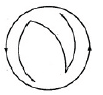Anti-clockwise current behaves like north pole.

Question. What are magnetic field lines? Justify the following statements
(a) Two magnetic field lines never intersect each other.
(b) Magnetic field lines are closed curves.
Answer :  Magnetic field lines: It is defined as the path along which the unit North pole (imaginary) tends to move in a magnetic field if free to do so.

(a) The magnetic lines of force do not intersect (or cross) one another. If they do so then at the point of intersection, two tangents can be drawn at that point which indicates that there will be ,two different directions of the same magnetic which field, i.e. the compass needle points in two different directions which is not possible.
(b) Magnetic field lines are closed continuous curves. They diverge from the north pole of a bar magnet and converge its south pole. Inside the magnet they move from south pole to north pole.

Question. Why and when does a current carrying conductor kept in a magnetic field experience force? List the factors on which direction of this force depends?
Answer : The drifting of free electrons of a conductor in a definite direction causes the current to flow through it. When such conductor is placed in a uniform magnetic field, each drifted electron of a conductor experience a magnetic force. This force is collectively experience by a conductor as a whole. Hence a current carrying conductor kept in a magnetic field experience a force. The direction of magnetic force depends on
(i) direction of current through the conductor, and
(ii) direction of magnetic field.

Question.Which of the following property of a proton can change while it moves freely in a magnetic field.
(There may be more than one correct answer.)
(i) Mass
(ii) Speed
(iii) Velocity
(iv) Momentum
Answer : The Choose the correct option: (iii) velocity, (iv) momentum.

Question. The phenomenon of electromagnetic induction is
(i) the process of charging a body
(ii) the process of generating magnetic field due to a current passing through a coil
(iii) producing induced current in a coil due to relative motion between a magnet and the coil
(iv) the process of rotating a coil of an electric motor
(iii) Producing induced current in a coil due to relative motion between a magnet and the coil

Question.What is meant by solenoid? How does a current carrying solenoid behave? Give its main use.
Answer : Solenoid: A coil of many circular turns of insulated copper wire wound on a cylindrical insulating body (i.e., cardboard etc.) such that its length is greater than its diameter is called solenoid.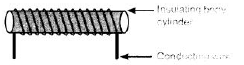When current is flowing through the solenoid, the magnetic field line pattern resembles exactly with those of a bar magnet with the fixed polarity,
i.e. North and South pole at its ends and it acquires the directive and attractive properties similar to bar magnet. Hence, the current carrying solenoid behave as a bar magnet.
Use of current carrying solenoid: It is used to form a temporary magnet called electromagnet as well as permanent magnet.

Question a)Describe an activity to demonstrate the pattern of magnetic field lines around a straight conductor carrying current.
(b) State the rule to find the direction of magnetic field associated with a current carrying conductor.
(c) What is the shape of a current carrying conductor whose magnetic field pattern resembles that of a bar-magnet ?
(a) Aim : To study the magnetic field due to a straight current carrying conductor.
Apparatus Required : A thick conducting wire, battery, rheostat, magnetic needle, ammeter (0-5 A), key, a cardboard, a stand to hold the wire, iron filings and sprinkler of iron filings.
Procedure :
1. Attach the thick wire through a hole at the middle of the cardboard and clamp it in a stand.
2. Attach the ends of the wire through a key, variable resistor and an ammeter on either side of a battery and hold it vertically and perpendicularly to the board.

3. Spread the iron filings uniformly on the cardboard and place the magnetic needle on the board.
4. Close the key and tap the cardboard slightly and observe the orientation of iron filings.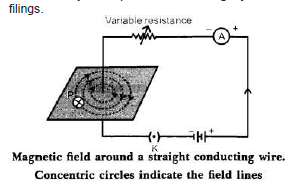Observation :
1. Just on closing the key, the iron filings are aligned in the pattern of concentric circles around the wire.

Conclusion :
1. Current carrying conductor is a source of magnetic field.
2. The magnetic field is in the form of concentric circles whose centre lies on the wire.
(b) Right-Hand Thumb Rule. This rule is used to find the direction of magnetic field due to a straight current carrying wire.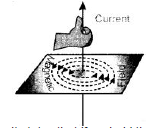It states that if we hold the current carrying-conductor in the right hand in such a way that the thumb is stretched along the direction of current, then the curly finger around the conductor represent the direction of magnetic field produced by it. This is known as right-hand thumb rule.
Direction of Field Lines due to current carrying straight conductor is as shown in figure.

(c) Solenoid.

 CBSE Class 10 Science Chemical Reactions and Equations Assignment
 CBSE Class 10 Science Acids Bases and Salts Assignment Set A CBSE Class 10 Science Acids Bases and Salts Assignment Set B CBSE Class 10 Science Acids Bases and Salts Assignment Set C
 CBSE Class 10 Science Metals and Non Metals Assignment Set A CBSE Class 10 Science Metals and Non Metals Assignment Set B
 CBSE Class 10 Science Carbon and its compounds Assignment Set A CBSE Class 10 Science Carbon and its compounds Assignment Set B CBSE Class 10 Science Carbon and its compounds Assignment Set C CBSE Class 10 Science Carbon and its compounds Assignment Set D CBSE Class 10 Science Carbon and its compounds Assignment Set E
 CBSE Class 10 Science Periodic Classification Of Elements Assignment Set A CBSE Class 10 Science Periodic Classification Of Elements Assignment Set B CBSE Class 10 Science Periodic Classification Of Elements Assignment Set C
 CBSE Class 10 Science Biology Life Process Respiration Assignment CBSE Class 10 Science Biology Nutrition Assignment CBSE Class 10 Science Biology Respiration Assignment CBSE Class 10 Science Biology Transport Enrichment Assignment CBSE Class 10 Science Biology Transportation Assignment CBSE Class 10 Science Life Processes Assignment Set A CBSE Class 10 Science Life Processes Assignment Set B CBSE Class 10 Science Life Processes Assignment Set C
 CBSE Class 10 Science Control and Coordination Assignment Set A CBSE Class 10 Science Control and Coordination Assignment Set B CBSE Class 10 Science Control and Coordination Assignment Set C CBSE Class 10 Science Control and Coordination Assignment Set D
 CBSE Class 10 Science How do Organisms Reproduce Assignment Set A CBSE Class 10 Science How do Organisms Reproduce Assignment Set B
 CBSE Class 10 Science Heredity and Evolution Assignment Set A CBSE Class 10 Science Heredity and Evolution Assignment Set B CBSE Class 10 Science Heredity and Evolution Assignment Set C
 CBSE Class 10 Science Light Reflection and Refraction Assignment Set A CBSE Class 10 Science Light Reflection and Refraction Assignment Set B CBSE Class 10 Science Light Reflection and Refraction Assignment Set C CBSE Class 10 Science Light Reflection and Refraction Assignment Set D CBSE Class 10 Science Light Reflection and Refraction Assignment Set E CBSE Class 10 Science Light Reflection and Refraction Assignment Set F
 CBSE Class 10 Science Human Eye and Colorful World Assignment Set A CBSE Class 10 Science Human Eye and Colorful World Assignment Set B CBSE Class 10 Science Human Eye and Colorful World Assignment Set C
 CBSE Class 10 Physics Electricity Assignment Set A CBSE Class 10 Physics Electricity Assignment Set B CBSE Class 10 Physics Electricity Assignment Set C CBSE Class 10 Science Electricity Assignment Set A CBSE Class 10 Science Electricity Assignment Set B CBSE Class 10 Science Electricity Assignment Set C
 CBSE Class 10 Science Magnetic Effects of Electric Current Assignment Set A CBSE Class 10 Science Magnetic Effects of Electric Current Assignment Set B CBSE Class 10 Science Magnetic Effects of Electric Current Assignment Set C CBSE Class 10 Science Magnetic Effects of Electric Current Assignment Set D
 CBSE Class 10 Science Sources of Energy Assignment Set A CBSE Class 10 Science Sources of Energy Assignment Set B CBSE Class 10 Science Sources of Energy Assignment Set C CBSE Class 10 Science Sources of Energy Assignment Set D
 CBSE Class 10 Science Our Environment Assignment Set A CBSE Class 10 Science Our Environment Assignment Set B CBSE Class 10 Science Our Environment Assignment Set C
 CBSE Class 10 Science Sustainable Management of Natural Resources Assignment Set A CBSE Class 10 Science Sustainable Management of Natural Resources Assignment Set B CBSE Class 10 Science Sustainable Management of Natural Resources Assignment Set C
 CBSE Class 10 Physics Revision Assignment Set A CBSE Class 10 Physics Revision Assignment Set B CBSE Class 10 Physics Revision Assignment Set C CBSE Class 10 Physics Revision Assignment Set D CBSE Class 10 Physics Revision Assignment Set E CBSE Class 10 Physics Revision Assignment Set F CBSE Class 10 Physics Revision Assignment Set G CBSE Class 10 Physics Revision Assignment Set H
 CBSE Class 10 Science Assignment CBSE Class 10 Science Assignments Collection CBSE Class 10 Science Energy Crossword Puzzle Assignment
 CBSE Class 10 Physics Question Bank CBSE Class 10 Physics Tungsten Assignment
 CBSE Class 10 Science Chemistry Cleaning Capacity of Soap Assignment CBSE Class 10 Science Chemistry Determination of pH Assignment CBSE Class 10 Science Chemistry Different Types of Chemical Reactions Assignment CBSE Class 10 Science Chemistry Displacement Reaction and Reactivity Series Assignment CBSE Class 10 Science Chemistry Properties of Acetic Acid Assignment CBSE Class 10 Science Chemistry Saponification Preparation of Soap Assignment CBSE Class 10 Science Chemistry Study of properties of HCI Acid and NaOH Assignment

## More Study Material

Tags

### CBSE Class 10 Science Chapter 13 Magnetic Effect Of Electric Current Assignment

We hope you liked the above assignment for Chapter 13 Magnetic Effect Of Electric Current which has been designed as per the latest syllabus for Class 10 Science released by CBSE. Students of Class 10 should download and practice the above Assignments for Class 10 Science regularly. We have provided all types of questions like MCQs, short answer questions, objective questions and long answer questions in the Class 10 Science practice sheet in Pdf. All questions have been designed for Science by looking into the pattern of problems asked in previous year examinations.

#### Assignment for Science CBSE Class 10 Chapter 13 Magnetic Effect Of Electric Current

Our team of expert teachers have referred to NCERT book for Class 10 Science to design the Science Class 10 Assignments. If you practice at least one test paper daily, you will get higher marks in Class 10 exams this year. Daily practice of Science course notes and related study material will help you to clear all your doubts and have stronger understanding of all concepts. You can download all Revision notes for Class 10 Science also from www.studiestoday.com absolutely free of cost.

#### Chapter 13 Magnetic Effect Of Electric Current Assignment Science CBSE Class 10

All questions and their answers for the assignment given above for Class 10 Science have been developed as per the latest curriculum and books issued for the current academic year. The students of Class 10 can rest assured that the best teachers have designed the questions of Science so that you are able to revise the entire syllabus if you do the assignments. Lot of MCQ questions for Class 10 Science have also been given in the worksheets and assignments for regular use. All study material for Class 10 Science students have been given on studiestoday.

#### Chapter 13 Magnetic Effect Of Electric Current Assignment CBSE Class 10 Science

Regular assignment practice helps to get a more comprehensive understanding of Chapter 13 Magnetic Effect Of Electric Current concepts. Assignments play a crucial role in understanding Chapter 13 Magnetic Effect Of Electric Current in CBSE Class 10. Students can download all the assignments of the same chapter in Class 10 Science in Pdf format. You can print them or read them online on your computer or mobile.

#### CBSE Science Class 10 Chapter 13 Magnetic Effect Of Electric Current Assignment

CBSE Class 10 Science latest books have been used for coming up with the latest questions and solutions for the above assignment. If you have revised all concepts relating to Chapter 13 Magnetic Effect Of Electric Current then you should attempt all questions given in the test sheets above. We have also provided lot of Worksheets for Class 10 Science which you can use to further make your self stronger in Science

Where can I download in PDF assignments for CBSE Class 10 Science Chapter 13 Magnetic Effect Of Electric Current

You can download free Pdf assignments for CBSE Class 10 Science Chapter 13 Magnetic Effect Of Electric Current from StudiesToday.com

The assignments for Chapter 13 Magnetic Effect Of Electric Current Class 10 Science for have been made based on which syllabus

The Chapter 13 Magnetic Effect Of Electric Current Class 10 Science Assignments have been designed based on latest CBSE syllabus for Class 10 Science issued for the current academic year

Can I download and print these printable assignments for Science Chapter 13 Magnetic Effect Of Electric Current Class 10

Yes, These printable assignments for Chapter 13 Magnetic Effect Of Electric Current Class 10 Science are free to download and print

How many topics are covered in Chapter 13 Magnetic Effect Of Electric Current Science assignments for Class 10

All topics given in Chapter 13 Magnetic Effect Of Electric Current Science Class 10 Book for the current academic year have been covered in the given assignment

Is there any charge for this assignment for Chapter 13 Magnetic Effect Of Electric Current Science Class 10

No, all Printable Assignments for Chapter 13 Magnetic Effect Of Electric Current Class 10 Science have been given for free and can be downloaded in Pdf format

How can I download the printable test assignments for Chapter 13 Magnetic Effect Of Electric Current Science Class 10

Just click on the View or Download button below, then another window with the Pdf will be visible, just click on the Pdf icon to download the free assignments for Chapter 13 Magnetic Effect Of Electric Current Class 10 Science

Are these assignments available for all chapters in Class 10 Science

Yes, apart from Science you can download free assignments for all subjects in Class 10

Can I download solved assignments for Chapter 13 Magnetic Effect Of Electric Current CBSE Class 10 Science

Our team of expert teachers at studiestoday.com have provided all answers for the practice questions which have been given in Class 10 Science Chapter 13 Magnetic Effect Of Electric Current assignments